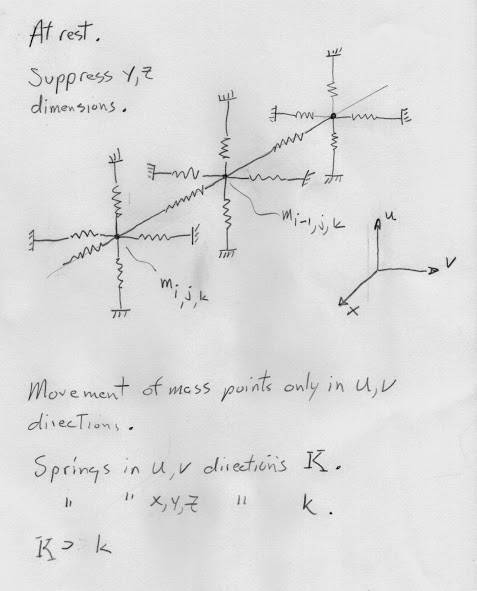# Masses and springs in R^3XR^2 and K.G. Eq. on a lattice?

Gold Member
Let us have a 5 dimensional lattice in R^5 = R^3XR^2, where at each lattice point we have a point mass and all mass points are linked with springs in all 5 dimensions (edit, to nearest neighbors). Require that motion of the mass points is restricted to a two dimensional subspace, R^2, of R^5. Further let the springs in R^2 be much stiffer then the remaining springs. Make further assumptions as required so the problem comes out right.

Edit, something does not smell right, should of thought more, think I am close but I think the above is wrong, damn.

Edit, we want only one mass point in the subspace R^2?

4th edit, we want a 3 dimensional mass spring lattice system with movement of the masses only in some 2 dimensional tangent space at each mass point and that movement is that of 2 dimensional harmonic osscilator coupled with springs to nearest neighbors in 3d? Put a 2d H.O. at each lattice point in 3d and couple the movement to nearest neighbors in 3d with springs? 5th edit, all done in a 5d space?

Is the lagrangian of this system similar to the lagrangian for the Klein Gordon equation on a lattice?

Is there a electrical circuit equavalent using capacitors and inductors of the above system?

If true can one expand on the ideas above and come up with a mass/spring system on some space whose lagrangian is the same as the lagrangian that gives the Dirac eq. on a lattice?

Let us ignor relativity (set c to infinity?).

Thanks for any help!

Last edited: